# AP Calculus AB Practice Test 52

### Test Information10 questions20 minutes

Calculator Disallowed

1.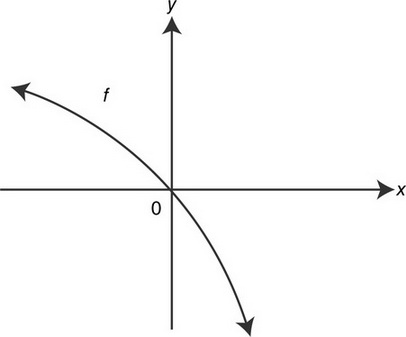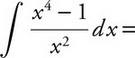2. If p′(x) = q(x) and qis a continuous function for all values of x, then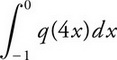is

3. Water is leaking from a tank at a rate represented by f(t) whose graph is shown below. Which of the following is the best approximation of the total amount of water leaked from the tank for 1 ≤ t≤ 3?

4.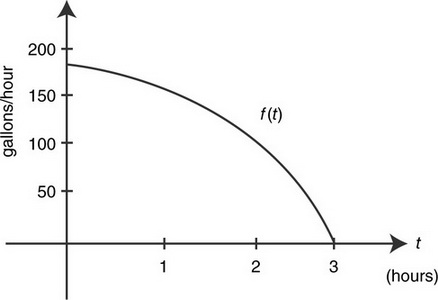If f(x) = 5 cos2(π&- x), then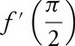is

5.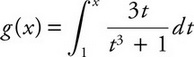, then g′(2) is

6. If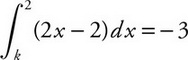, a possible value of kis

7. If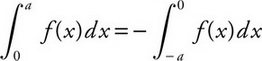for all positive values of a, then which of the following could be the graph of f? (See below.)

8. A function fis continuous on [1, 5] and some of the values of fare shown below: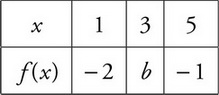If fhas only one root, r, on the closed interval [1, 5], and r≠ 3, then a possible value of bis

9. Given the equation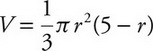, what is the instantaneous rate of change of Vwith respect to rat r= 5?

10. What is the slope of the tangent to the curve x3&- y2= 1 at x= 1?